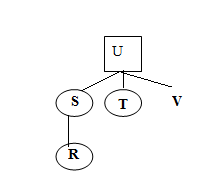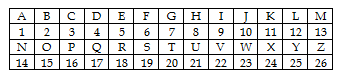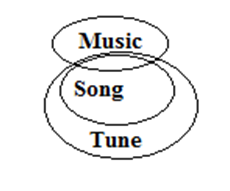# SSC CGL 2018 Practice Test Papers | Reasoning Ability (Day-64)

Dear Aspirants, Here we have given the Important SSC CGL Exam 2018 Practice Test Papers. Candidates those who are preparing for SSC CGL 2018 can practice these questions to get more confidence to Crack SSC CGL 2018 Examination.

[WpProQuiz 3855]

Click “Start Quiz” to attend these Questions and view Explanation

1) Select the odd number from given alternatives

a) 1600

b) 3600

c) 2500

d) 4000

2) The weight of 4 boxes is 80, 90, 70, and 60 kilograms. Which of the following cannot be the total weight, in kilograms of any combination of these boxes?

a) 200

b) 210

c) 300

d) 220

3) In the following series, select the missing number from the given series.

3, 7, 16, 35, ?

a) 85

b) 79

c) 74

d) 89

4)  If A 3 B means ‘A is daughter of B’, A 5 B means ‘ A is father of B’, A 7 B means ‘A is mother of B’, A 9 B means ‘ A is sister of B’, then how S is related to V in R 3 S 9 T 3 U 5 V ?

a) Mother

b) Father

c) Sister

d) Brother

5) In the following, from the given alternatives, select the word which cannot be formed using the letters of the given word.

FLABBERGASTED

a) FABULOUS

b) FLAGSTAR

c) BLASTED

d) TEASER

6) In the certain code language, “DAD” is written as “8” and “SAI” is written as “2”. How VAN is written in the code language?

a) 0

b) 1

c) 2

d) 3

7) If 4*5 % 3 = 8000 and 2 * 3 % 2 = 36, then 4 * 3 % 3 = ?

a) 3375

b) 4096

c) 1000

d) 1728

Direction (8 – 9): In each of the following question below are given some statements followed by some conclusions. Taking the given statements to be true even if they seem to be at variance from commonly known facts, read all the conclusions and then decide which of the given conclusion logically follows the given statements.

8) Statements

Some music is song

All song is tune

Conclusion

1. No tune is Music
2. Some music is Tune

a) Only I follow

b) Only II follow

c) Either I or II follow

d) Neither I nor II follow

9) Statements

Some music is song

All song is tune

Conclusion:

1. Some Tune is Music
2. All tunes is Music

a) Only I follow

b) Only II follow

c) Either I or II follow

d) Neither I nor II follow

10) In the following question, select the odd letters from the alternatives.

a) 73

b) 61

c) 111

d) 97

4000 is not a Square number.

80 + 70 + 60 = 210

80 + 90 + 70 + 60 = 300

60+90+70 = 220

3 * 2 + 1 = 7

7 * 2 + 2 = 16

16 * 2 + 3 = 35

35 * 2 + 4 = 74FABULOUS cannot be formed using FLABBERGASTEDDAD = 4 + 1 + 4 = 9

SAI = 19 + 1 + 9 = 29 = 2 + 9 = 11 = 1 + 1 = 2

VAN = 22 + 1 + 14 = 37 = 3 + 7 = 10 = 1 + 0 = 1

4*5 % 3 = (4× 5)3 = 203 = 8000

2 * 3 % 2 = (2×3)2 = 62 = 63

4 * 3 % 3 = (4× 3)3 = 123 = 1728

Direction (8-9) :